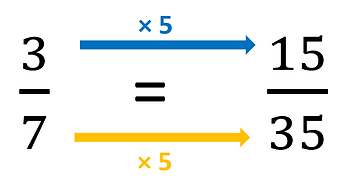top of page

## Topic 16: Ordering fractions, decimals and percentagesYou may be asked to put a list of fractions in order of value. Here is an example of a list of fractions to be ordered.

One way to be sure about the correct order is to convert all of the fractions into decimals (see Topic 15 on conversion).

1/3    = 1 ÷ 3    = 0.333...

3/8    = 3 ÷ 8    = 0.375

1/6    = 1 ÷ 6    = 0.1666...

2/5    = 2 ÷ 5    = 0.4

So,   2/5  >  3/8  >  1/3  >  1/6

If converting to decimals is too difficult without a calculator, you may need to use another method.

You may be uncertain about which of two fractions is the greater, because they have different denominators, you can produce equivalent fractions with common denominators.

For example, it may not be immediately obvious which of these fractions is greater:

To give both fractions the same denominator, multiply the two denominators together. 7 × 5 = 35.

Find equivalents for both fractions that have the denominator 35.

This helps to show that 3/7 is greater than 2/5bottom of page